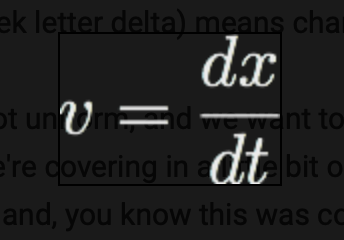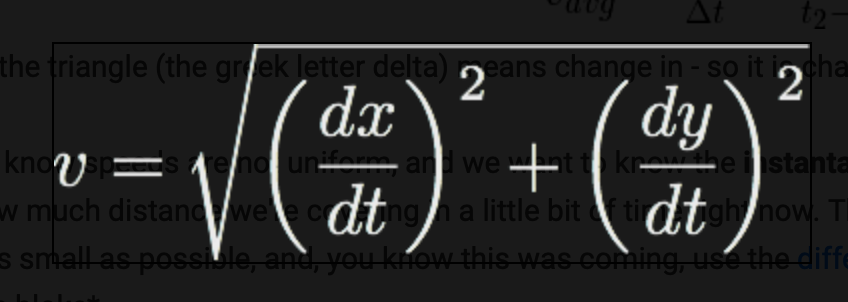Search

The only thing that isn't moving at the speed of light is light itself!

A repost from 2013! Relativity purists will grumble over some of what is written here, but it address the key point. You are always moving at the speed of light!

Time to give you some brain food. The title of this post is correct, and I will go further.

"and the speed that light travels is not the speed of light, it is zero"

I hope that this has opened your eyes and got the cog-wheels turning. You might be even thinking that I have gone slightly mad. But stick with me, and you'll see that this is true, and you can use these facts to wow your mates down the pub. The key issue is the difference of traveling through space, and traveling through space-time. I think the latter is more fundamental, and so my statement is more truerer than the usual comments you hear. But to understand this, we need to remember what speed actually is! Remember from school that speed is simply the distance travelled divided by the time that it took to travel the distance. If we moving at uniform speed, then our speed at all points in the journey would be the distance covered divided by the time. Maths time!Where the triangle (the greek letter delta) means change in - so it is change in x (distance) over change in t (time). But we know speeds are not uniform, and we want to know the instantaneous speed. This means that we want to find out just how much distance we're covering in a little bit of time right now. This means that we need to take the delta up there to be as small as possible, and, you know this was coming, use the differential form, invented by Newton and some german bloke*. This means that the speed is just the slope of the plot of distance verses time, something like this:and we write the speed as:Excellent, now we have speed in one direction. But what if we are moving in two directions, x and y. What's our speed then? Remembering your high-school physics, we could talk about the vector components of the velocity, and the speed would be the magnitude of the vector, so we would haveClearly, if I am not moving, then the velocity components are zero, and so is my speed, so what the flip was I talking about at the beginning of this post? OK, we need to move from the concept of motion through space to movement though space-time. This is now motion through 4-D, three spatial dimensions and one time dimension. We have a picture that looks like thisJust as above, we can define a velocity through space-time defining the tangent to the "world-line", the path we trace out. But we have a little bit of a problem; looking back, the derivative is with respect to time (i.e. the change of distance over time), but time is now one of the dimensions. This is where it gets cool. There is another time involved, and that's the time that ticks off on watch as I trundle along my world-line. This is called the proper time and is denoted by the greek symbol τ, and, of course, runs at a different rate when compared to a clock at rest in the coordinate system. If we take our derivatives with respect to this proper-time, and then ask the question "What is the speed through space-time?" we getWhile this looks similar the equation above for the speed, there are some important differences. The first are the minus signs in there. For those interested in the technical reason, it's because space-time is pseudo-Riemannian, and a simple application of Pythagorous's theorem does not apply. If you are not interested in the technical, just take it that space-time is weird. However, these minus signs have an interesting effect (well, truth be told, all of the interesting effects in relativity are due to these minus signs). Firstly, it means that the right-hand side of the equation is one - we'll come back to this in a moment. But as the velocity in through space changes, the velocity through time changes accordingly, so that we always get one on the right-hand side. But this you knew; special relativity says that the relative rate that clocks tick depends upon the velocity. Back to what the right-hand side means. Well, it's the speed through space-time, and it's a constant. And in the units I've adopted here, this one is the speed of light. Your motion through space-time is the speed of light. It is always the speed of light, even when you are standing still. How cool is that!! Now, this works for any massive object. If someone tells you what you a lazing away, sleeping on the couch, point out that you are moving at the speed of light and tell them to leave you alone. Although, I am not sure you can use this information to beat a speeding ticket! Right, time to get ready for work, but what about non-massive objects, namely light. Well, things are trickier, as light does not have a proper time we can take the derivatives with respect to, but there is another thing (which, if you must know, is called an affine parameter) with which we can calculate the speed through space-time. This now looks like this;As you can see, the derivatives look very similar, but the right-hand side is now zero! The speed that light travels through space-time is zero! Welcome to the wonderfully whacky world of relativity. * Apologies to my German colleagues - I know perfectly well how much Leibniz contributed to the development of differential calculus, and I know how much Newton's ego influenced the history of the discovery.